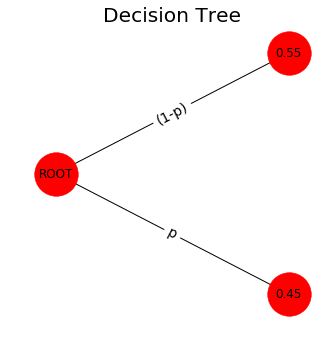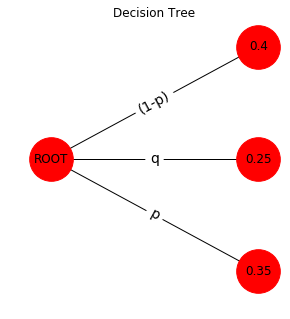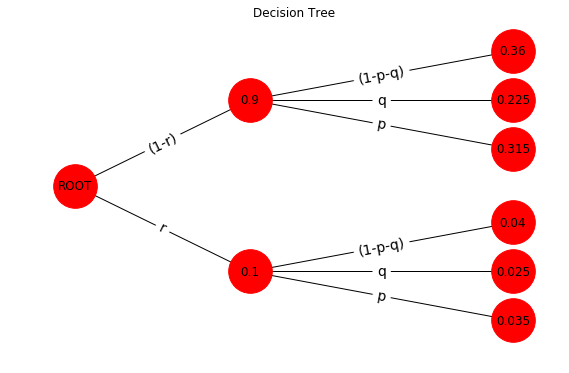.

.

.

.

.

# HealthyNumerics

HealthPoliticsEconomics | Quant Analytics | Numerics

# MetaAnalysis 02: A Medical Decision Tree Intro

## Decision tree for state of health

We denote different states of health, e.g., healthy, ill, dead, with $$H^h, H^i, H^d$$. We can assume that $$H^i$$ can be any health state bewteen $$H^h$$ and $$H^d$$. But this choice is not mandatory. We can choose any state of health $$H^A, H^B, H^C$$.

Let's assume an individual has at time $$t_o$$ a health state $$i$$, which is denoted as $$H_0^i$$. The individual may be confronted with the choice between $$H_0^i$$ and the uncertain prospect $$[H_1^h, H_1^d]^T$$ or $$[H_1^h, H_1^i, H_1^d]^T$$ due to a therapy. $$p$$, $$q$$, and $$(1-p-q)$$ are the probablities for the different outcomes. A decision tree is concerned with the problem of changing the state of health from $$\mathbf{H}_0$$ to $$\mathbf{H}_1$$ including the uncertainities of the different outcomes.

## The 2-states model of a therapy

A simpler model may comprise two states of health as prospect of a therapy $$T$$

$$\begin{equation} \begin{array}{rcl} H_0^i & \xrightarrow[]{T} & \left[\begin{matrix} H^h_1\\ H^d_1\end{matrix}\right] \\ \end{array} \end{equation}$$

By summarizing the probablilties of the different outcomes as

$$\begin{equation} \begin{array}{rcl} \mathbf{p} & = & \left[\begin{matrix}p & (1-p)\end{matrix}\right] & = & diag(\mathbf{P}) \end{array} \end{equation}$$

we can form the operator as matrix $$\mathbf{P}$$

$$\begin{equation} \begin{array}{rcl} \mathbf{P}& = & \left[\begin{matrix}p & 0\\ 0 & (1-p) \end{matrix}\right] \end{array} \end{equation}$$

and we can now write the decision tree as a Markov chain model with one time step as

$$\begin{equation} \begin{array}{rcl} H_0^i & \xrightarrow[]{T} & \mathbf{P}\cdot\mathbf{H}_1 \\ & & = \left[\begin{matrix}p \cdot H^h_1\\ (1-p) \cdot H^d_1\end{matrix}\right] \end{array} \end{equation}$$

With $$\mathbf{p} = \left[ \begin{matrix}p & (1-p)\end{matrix} \right] = \left[ \begin{matrix}0.45 & 0.55\end{matrix} \right]$$ this model has this tree:

tree_2()## The 3-states model

$$\begin{equation} \begin{array}{rcl} H_0^i & \xrightarrow[]{T} & \mathbf{H}_1 = \left[\begin{matrix}H^h_1 \\ H^i_1 \\H^d_1\end{matrix}\right] \\ & \textrm{with} & \mathbf{p} = \left[\begin{matrix}p & q & (1-p-q)\end{matrix}\right] & = & diag(\mathbf{P})\\ & \textrm{and} & \mathbf{P} = \left[\begin{matrix}p & 0 & 0 \\ 0 & q & 0 \\ 0 & 0 & (1-p-q)\end{matrix}\right] \\ \end{array} \end{equation}$$

The decison tree as a Markov chain model with one time step is

$$\begin{equation} \begin{array}{rcl} H_0^i & \xrightarrow[]{T} & \mathbf{P}\cdot\mathbf{H}_1 \\ & & = \left[\begin{matrix}p \cdot H^h_1\\ q \cdot H^i_1 \$$1-p-q) \cdot H^d_1\end{matrix}\right] \end{array} \end{equation} With \mathbf{p} = \left[\begin{matrix}p & q &(1-p-q)\end{matrix}\right] = \left[\begin{matrix}0.35 & 0.25 & 0.40\end{matrix}\right] this model has this tree: tree_3()## A 2-level model of a compounded therapy or diagnosis If a diagnostic test and/or a therapeutical intervention is compounded by two distinguishable steps the model becomes: \begin{equation} \begin{array}{rcl} H_0^i & \xrightarrow[]{T_1} & \mathbf{P}\cdot\mathbf{H}_1 & \xrightarrow[]{T_2} &\mathbf{R} \cdot (\mathbf{P}\cdot\mathbf{H}_1) \end{array} \end{equation} With \begin{equation} \begin{array}{rcl} \mathbf{p} & = & \left[\begin{matrix}p & q &(1-p-q)\end{matrix}\right] & = & \left[\begin{matrix}0.35 & 0.25 & 0.40\end{matrix}\right] \\ \mathbf{r} & = & \left[\begin{matrix}r & (1-r)\end{matrix}\right] & = & \left[\begin{matrix}0.10 & 0.90\end{matrix}\right] \end{array} \end{equation} The decision tree has now the following form: tree_23()#### Evaluation of the tree On right side of the tree are 6 end points, called foils. A branche is the connection between a foil and the root. On each of this 6 branches the probabilities of p and r are multiplied. In order to evaluate all probablities of the foils one can imagine that p and r build a grid that can be computed by the outer product as demonstrated here and here in a different context. The matrix \(\mathbf{\Pi}$$ with the probabilities of the foils can be computed by$$ \begin{equation} \begin{array}{rcl} \mathbf{\Pi} & = & \mathbf{r}^T \cdot\mathbf{p} & = &\left[\begin{matrix}r \\ (1-r)\end{matrix}\right] \cdot \left[\begin{matrix}p & q &(1-p-q)\end{matrix}\right]\\ & & & = & \left[\begin{matrix}p r & q r & (1-p-q) r\\(1-r) p & (1-r) q & (1-p-q) (1-r)\end{matrix}\right] \end{array} \end{equation} $$Analytically this can be shown with SymPy, a Python library for symbolic mathematic import sympy as s s.init_printing() from IPython.display import display  p1,r1 = s.symbols("(1-p-q) (1-r)") p = s.Matrix([['p','q', p1]]) r = s.Matrix([['r', r1]]) T = r.T*p #outer product #display(p); display(r); display(T) $$\left[\begin{matrix}p r & q r & (1-p-q) r\\(1-r) p & (1-r) q & (1-p-q) (1-r)\end{matrix}\right]

Numerically this can be shown with the numpy function np.outer

#--- input --------
p = 0.35;  q = 0.25;   pn = np.array([ p, q,  1-p-q])
r = 0.10;              rn = np.array([ r, 1-r]      )
#--- outer product --------
Π  = np.outer(rn, pn)
print('Π =') ; print(Π)

Π =
[[ 0.035  0.025  0.04 ]
[ 0.315  0.225  0.36 ]]


All branches of the decision tree that connect the root with the foils are evaluatetd now.

### The Python code

import numpy as np
import networkx as nx
import matplotlib.pyplot as plt

def treeGrafics(G,figX,figY):
from IPython.core.pylabtools import figsize

with plt.style.context('fivethirtyeight'):
fig = plt.figure(figsize=(figX,figY))
pos=nx.get_node_attributes(G,'pos')
plt.title('Decision Tree')
nx.draw(G, pos, with_labels=True, arrows=False, node_size=1900)
edge_labels =dict([((u, v), d['label'])
for u, v, d in G.edges(data=True)])
nx.draw_networkx_edge_labels(G, pos, edge_labels=edge_labels,font_size=14)
plt.axis('off')
plt.show()

def setTriage_00(G,nodeIn,pn,ps,dx,dy,f,):
pos=nx.get_node_attributes(G,'pos')
pL = len(pn)
for i,pj in enumerate(pn):
ii = f*2*(i/(pL-1)-0.5) + dy
return G

def setTriage_11(G,nodeIn,pn,ps,dx,dy,f):
pos=nx.get_node_attributes(G,'pos')
pL = len(pn)
for i,pj in enumerate(pn):
ii = f*2*(i/(pL-1)-0.5) + dy
return G

def tree_2():
#--- input --------
p = 0.45;
figX = 4.5;  figY = 5

#--- compute tree ----------
pn = np.array([ p,    1-p]  )
ps = np.array(['p', '(1-p)'])

with plt.style.context('fivethirtyeight'):
G=nx.DiGraph()
pos=nx.get_node_attributes(G,'pos')
G = setTriage_00(G,"ROOT", pn,ps, 2, 0, 3.5)
treeGrafics(G,figX,figY)

def tree_3():
#--- input --------
p = 0.35; q = 0.25
figX = 4.5;  figY = 5

#--- compute tree ----------
pn = np.array([ p,  q,  1-p-q]  )
ps = np.array(['p','q','(1-p)'])

G=nx.DiGraph()
pos=nx.get_node_attributes(G,'pos')
G = setTriage_00(G,"ROOT", pn,ps, 2, 0, 3.5)
treeGrafics(G,figX,figY)

def tree_23():
#--- input --------
p = 0.35; q = 0.25
r = 0.10
figX = 9.5;  figY = 6

#--- compute tree ----------
pn = np.array([ p,  q,   1-p-q]  )
ps = np.array(['p','q','(1-p-q)'])

rn = np.array([ r,   1-r]  )
rs = np.array(['r','(1-r)'])

G=nx.DiGraph()